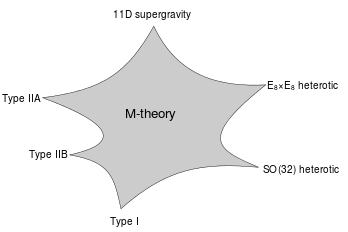# ２７　M-Theory

２７　M-Theory　【What is the Universe？】Dialogue with the Universe
[Electromagnetic force + Weak interaction＋Strong interaction ＋ Gravity]
1995 Edward Witten
○Hypothesis theory of 11-dimensional (spatial dimension is 10, one time dimension) comprehensive the five superstring theory that is currently known.
○In M-theory membrane of two-dimensional or 5-dimensional is considered as a component.
○While it is theoretically to be unified to represent the four force electromagnetic force, gravity, weak force, gravity in one form, this theory is the stage of mathematical hypothesis.
・・・We do not know how to quantize the membrane. Therefore, analysis of M theory is often done using eleven-dimensional supergravity theory which is low energy effective theory. 
○The M in M-theory can stand for "magic", "mystery", or "matrix" according to taste, and the true meaning of the title should be decided when a more fundamental formulation of the theory is known.※A schematic illustration of the relationship between M-theory, the five superstring theories, and eleven-dimensional supergravity. 

○In this theory, only gravitational field (graviton field) , gravitino field and another field are available.

【参　照】
1. M-Theory – Wikipedia

【Change log】